# 汽车与交通学院孟忠伟教授团队研究生黄俊峰在能源动力领域一区期刊上发表论文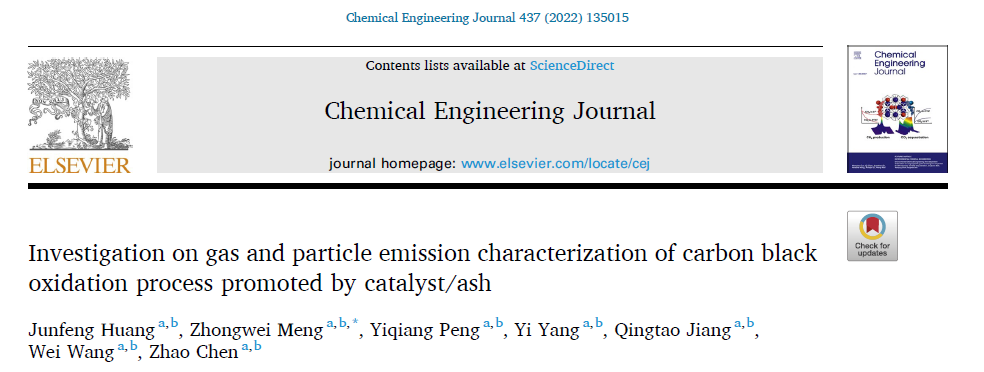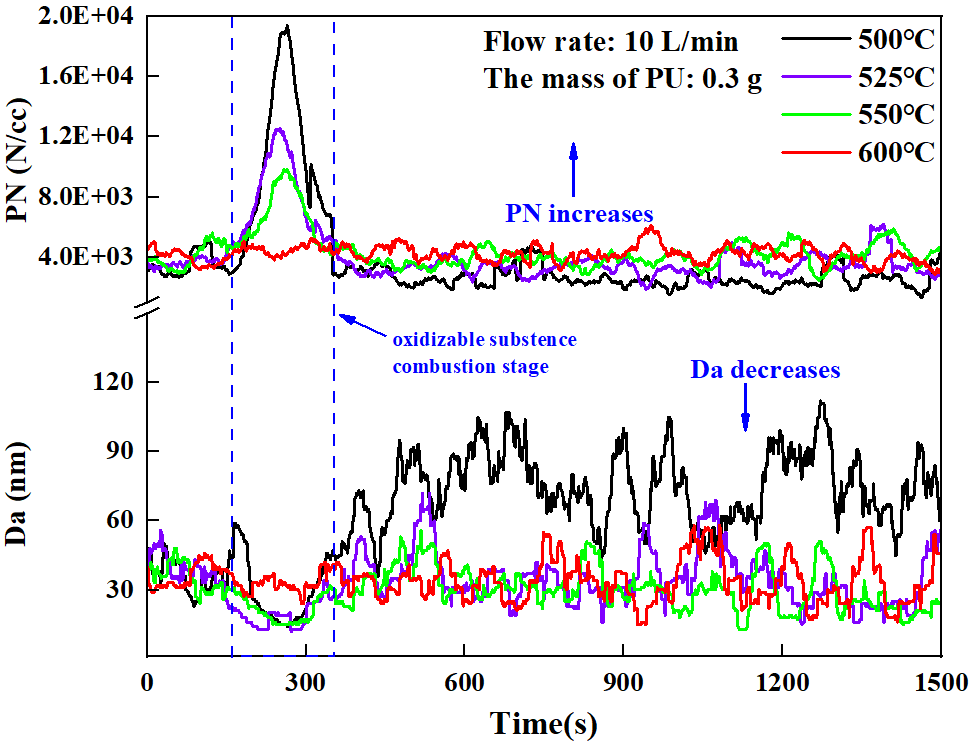Figure 1. Particle diameter concentration distribution diagram at different oxidation temperatures (heating oxidation stage)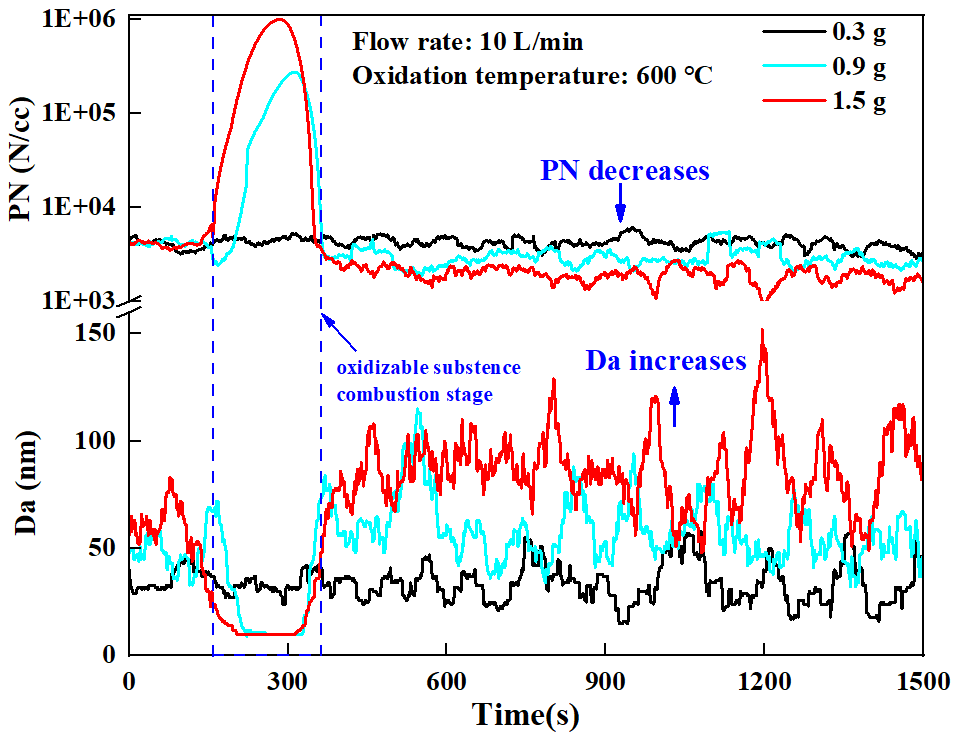Figure 2. Particle diameter concentration distribution diagram for different sample masses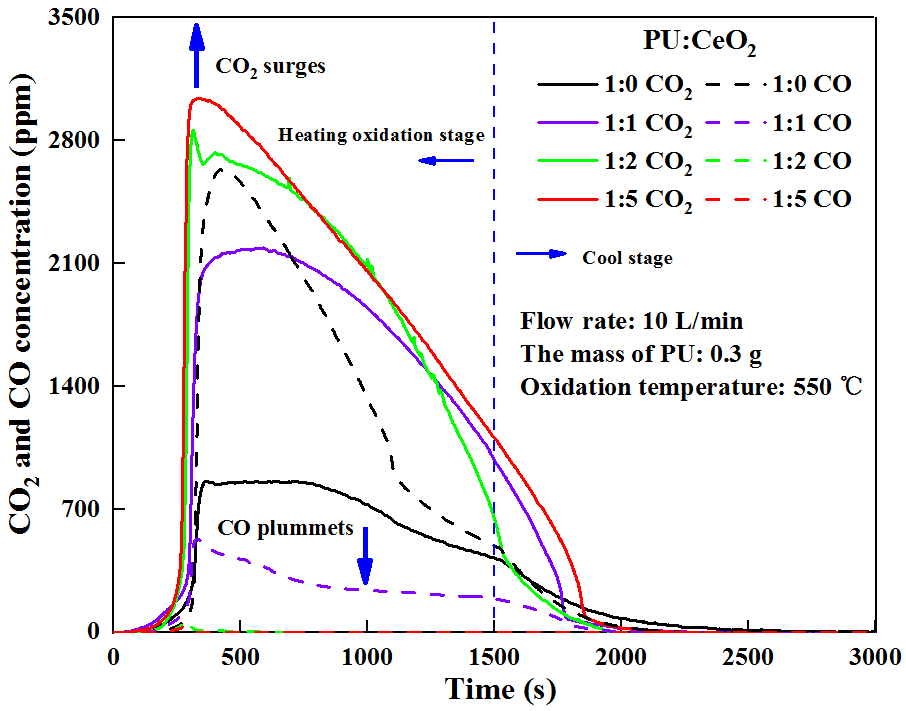Figure 3. PU oxidation gas emissions at different CeO2 ratios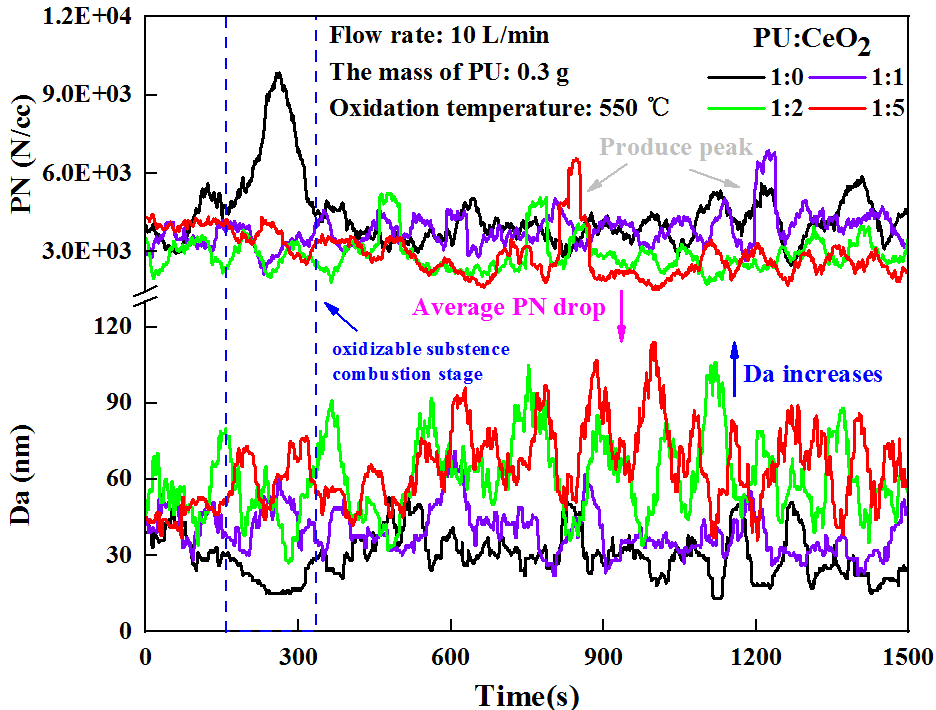Figure 4. Particle diameter concentration distribution diagram at different CeO2 ratios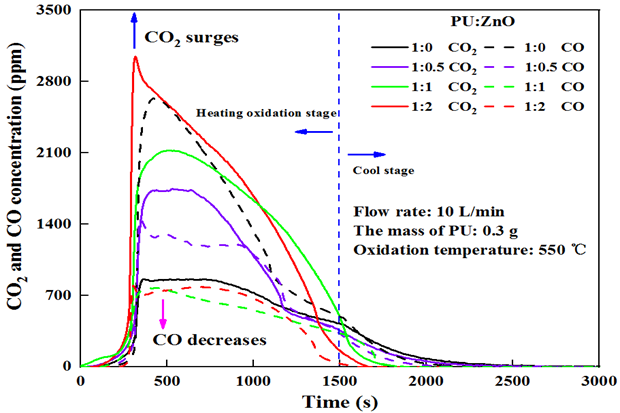Figure 5. PU oxidation gas emissions at different ZnO ratios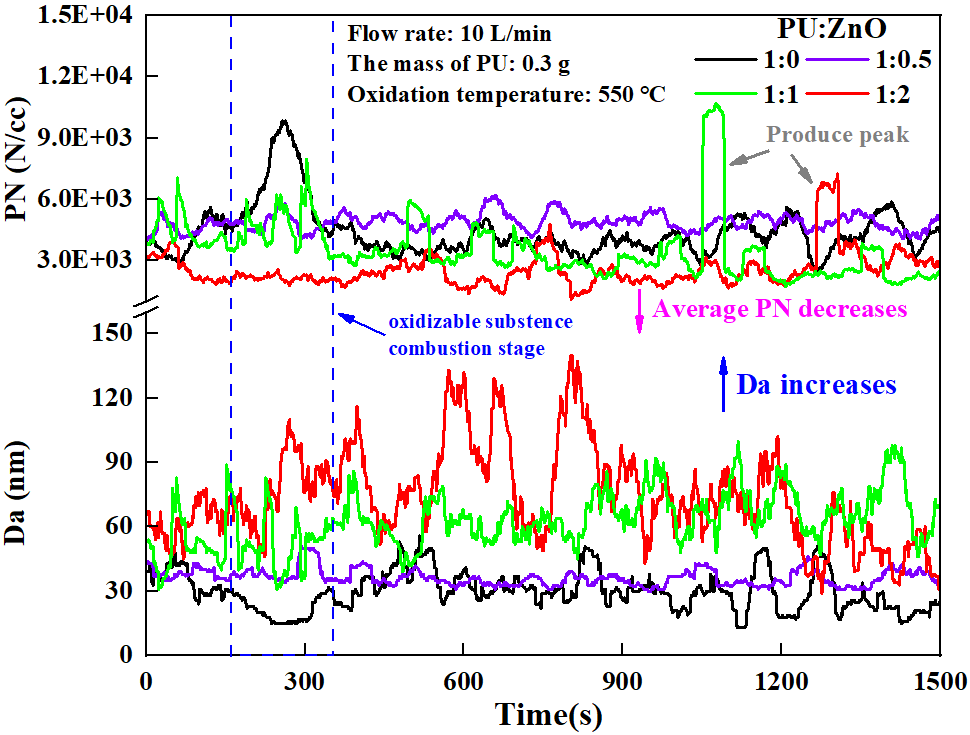Figure 6. Particle diameter concentration distribution diagram at different ZnO ratios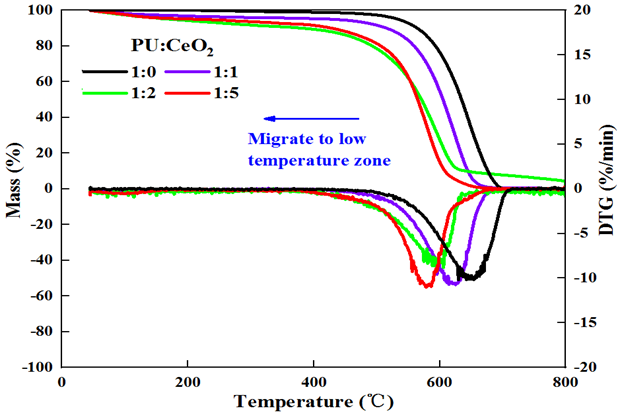Figure 7. TGA burning of PU at different CeO2 ratios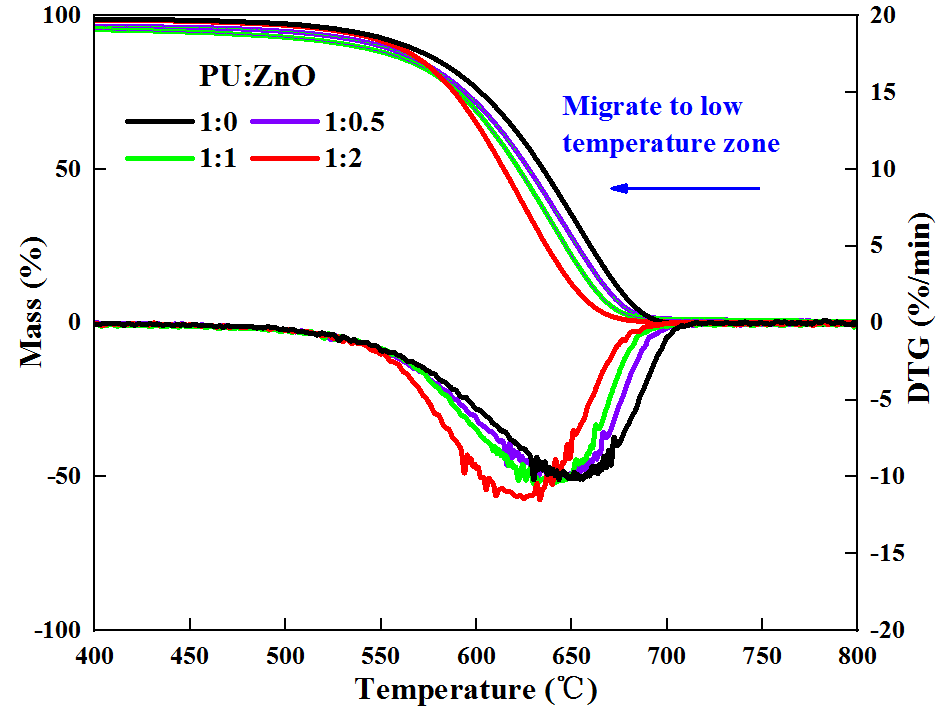Figure 8. TGA curve of PU at different ZnO ratios